Next: Tightly Wound Spirals Up: Galactic Scale Star Formation Previous: Local Star Formation Rate   Contents

# Gravitational Instability of Rotating Thin Disk

Here, we will derive the dispersion relation for the gravitational instability of a rotating thin disk. We will see the spatial variation of Toomre's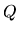parameter, which determines the stability of the rotating disk, explains the nonlinearity of star formation rate, that is, there is a threshold density and no stars are formed in the low density region. Use the cylindrical coordinate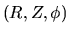and the basic equations for thin disk in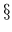2.6. In linear analysis, we assume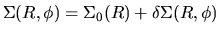,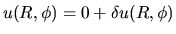,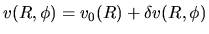, where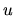and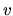represent the radial and azimuthal components of the velocity. Linearized continuity equation is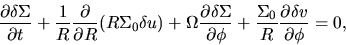(3.8)

where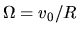. Linearized equations of motion are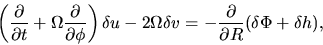(3.9)

and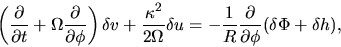(3.10)

where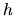is a specific enthalpy as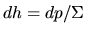and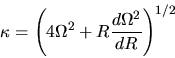(3.11)

is the epicyclic frequency. We assume any solution of equations (3.8), (3.9) and(3.10) can be written as a sum of terms of the form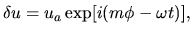(3.12)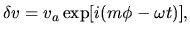(3.13)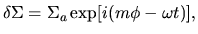(3.14)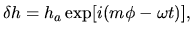(3.15)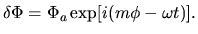(3.16)

Using the equation of state of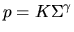,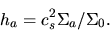(3.17)

Using equations (3.12)-(3.16), equations (3.8), (3.9), and (3.10) are rewritten as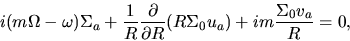(3.18)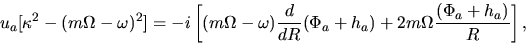(3.19)

and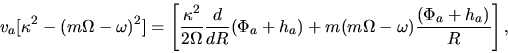(3.20)

SubsectionsNext: Tightly Wound Spirals Up: Galactic Scale Star Formation Previous: Local Star Formation Rate   Contents
Kohji Tomisaka 2007-07-08